# Program to check a Number is Negative or Positive

Input :-

Outputs : -

Code :-

#include <stdio.h>
#include <stdlib.h>

int main()
{
int a;

printf("Enter a number : \n");
scanf("%d", &a);
if
(a<0)
printf("The number is negative\n");
else if
(a==0)
printf("You Entered Zero\n");
else
printf("The number is positive\n");
return 0;

}

# To check a year is leap or not in C

To check a year is leap or not in C

Input :

Output :

Code :

#include <stdio.h>

int main()
{
int c;
printf("Enter the year :\n");
scanf("%d",&c);
{if ((c%4==0)&&(!(c%100==0)||(c%400==0)))
printf("The year is a leap year\n");
else
printf("The year is not a leap year\n");
}
return 0;
}

# To check whether a number is divisible by 6 and 4

To check whether a number is divisible by 6 and 4

Input :

Output :

Code :

#include <stdio.h>

int main()
{
int c;
printf("Enter a number :\n");
scanf("%d",&c);
{ if ((c%6==0) && (c%4==0))
printf("The number is divisible by 6 and 4\n");
else
printf("The number is not divisible by 6 and 4\n");
}
return 0;
}

# Program to print first 1000 number

Program to print first 1000 number

Input:-

Output:-

Code :-

#include <stdio.h>

int main()
{
int c=1;

while (c <= 1000)

{
printf("%d ", c);
c++;
}

return 0;
}

# To Check whether a number is prime or not

To Check whether a number is prime or not

Input:-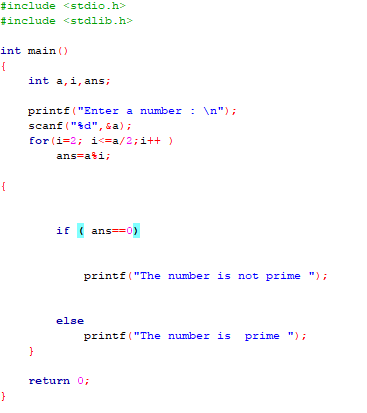Output:-

1)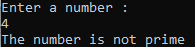2)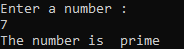Code:-

#include <stdio.h>
#include <stdlib.h>

int main()
{
int a,i,ans;

printf("Enter a number : \n");
scanf("%d",&a);
for(i=2; i<=a/2;i++ )
ans=a%i;

{

if ( ans==0)

printf("The number is not prime ");

else
printf("The number is  prime ");
}

return 0;
}

# Program to check divisibility by 5

Program to check divisibility by 5

Input: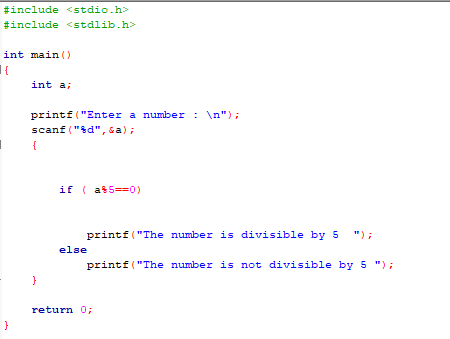Output:

1)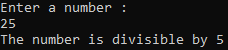2)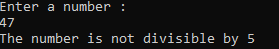Code:

#include <stdio.h>
#include <stdlib.h>

int main()
{
int a;

printf("Enter a number : \n");
scanf("%d",&a);
{

if ( a%5==0)

printf("The number is divisible by 5  ");
else
printf("The number is not divisible by 5 ");
}

return 0;
}

# Program to check a number is Even or Odd

Program to check a number is Even or Odd

Input :-Output :-

1)2)

Code :-

#include <stdio.h>
#include <stdlib.h>

int main()
{
int a;

printf("Enter a number : \n");
scanf("%d",&a);
{

if (a%2==0)
printf("The number is even\n\n");
else
printf("The number is odd\n\n");
}
return 0;

}

# C Program to check sum is less than or greater than hundred

C Program to check sum is less than or greater than hundred
Input :-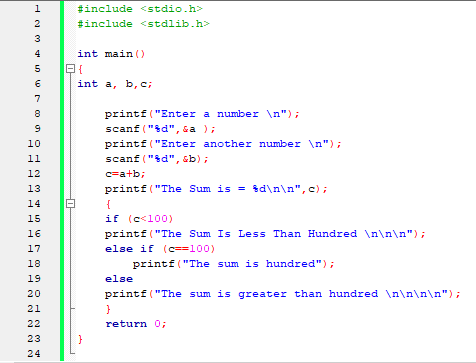Output :-
1)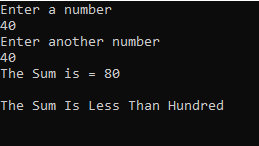2)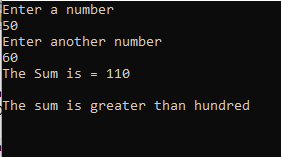3)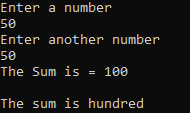Code :-

#include <stdio.h>
#include <stdlib.h>

int main()
{
int a, b,c;

printf("Enter a number \n");
scanf("%d",&a );
printf("Enter another number \n");
scanf("%d",&b);
c=a+b;
printf("The Sum is = %d\n\n",c);
{
if (c<100)
printf("The Sum Is Less Than Hundred \n\n\n");
else if (c==100)
printf("The sum is hundred");
else
printf("The sum is greater than hundred \n\n\n\n");
}
return 0;
}

# Lecture 53 Preincrement , PostIncrement Operators in C

Preincrement  , PostIncrement  Predecrement  , Postdecrement Operators in C

# Lecture 52 The C preprocessor

The C Preprocessor

# Lecture 51 Practices for writing larger programs in C

# Lecture 48 Pointers to Structures

# Lecture 47 Structures in C

Structures in C

# Lecture 46 Some other file handling functions

Some other file handling functions

## Categories

AI (1) Android (23) AngularJS (1) book (1) Books (36) C (76) C# (12) C++ (82) Course (51) Coursera (8) Data Science (19) Django (1) Downloads (3) edx (1) Engineering (13) Excel (1) flutter (1) FPL (17) Google (10) Hadoop (1) HTML&CSS (41) IBM (1) IS (25) Java (90) Leet Code (4) Meta (2) Pandas (2) PHP (20) Projects (26) Python (585) R (69) Software (15) SQL (28)